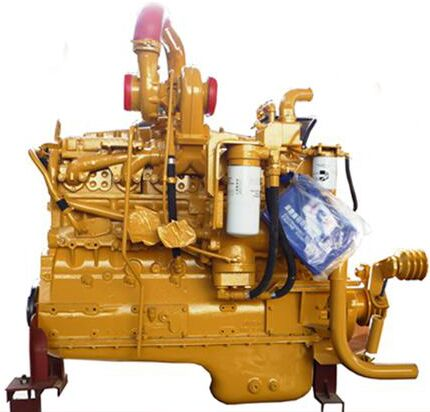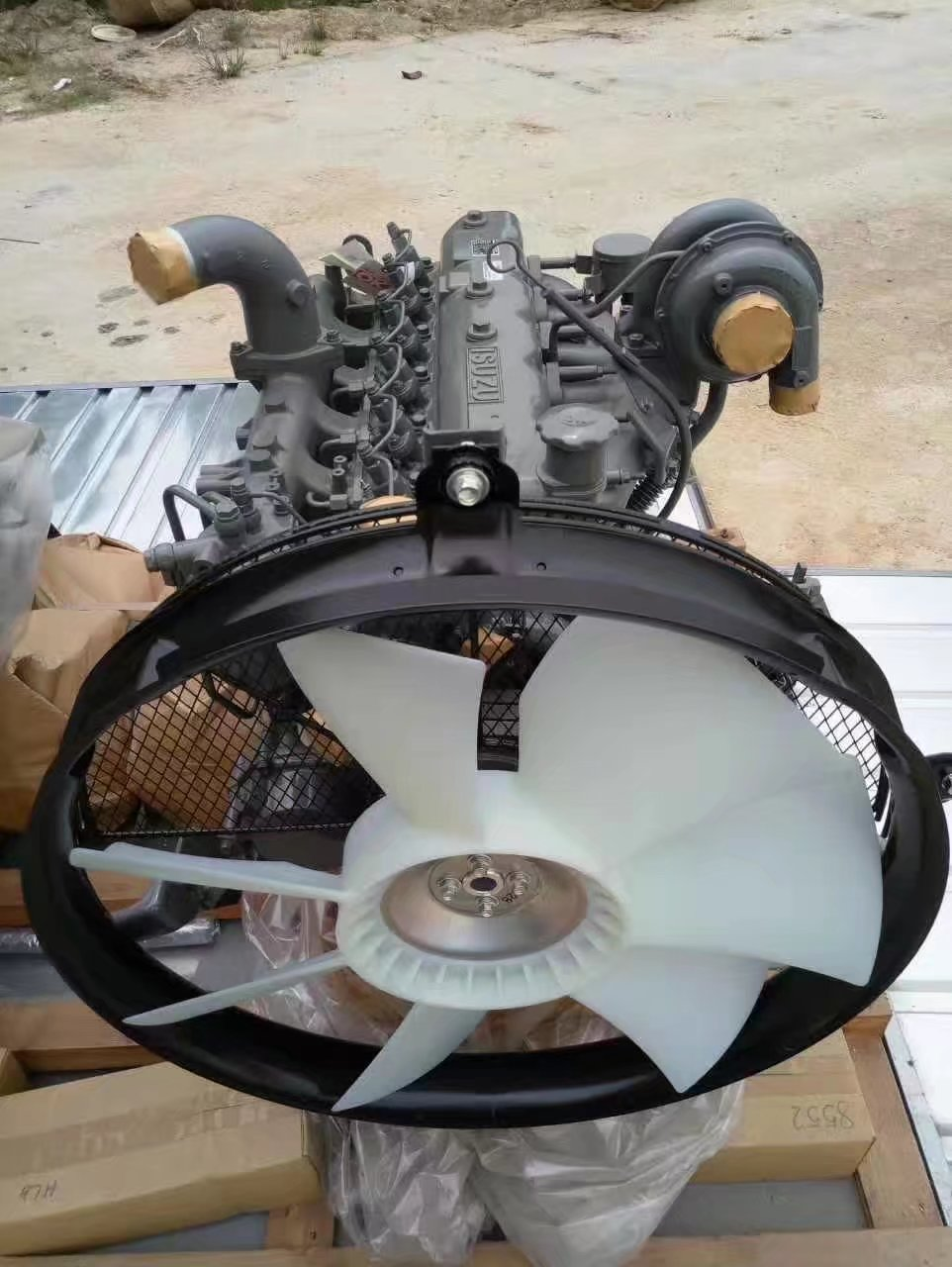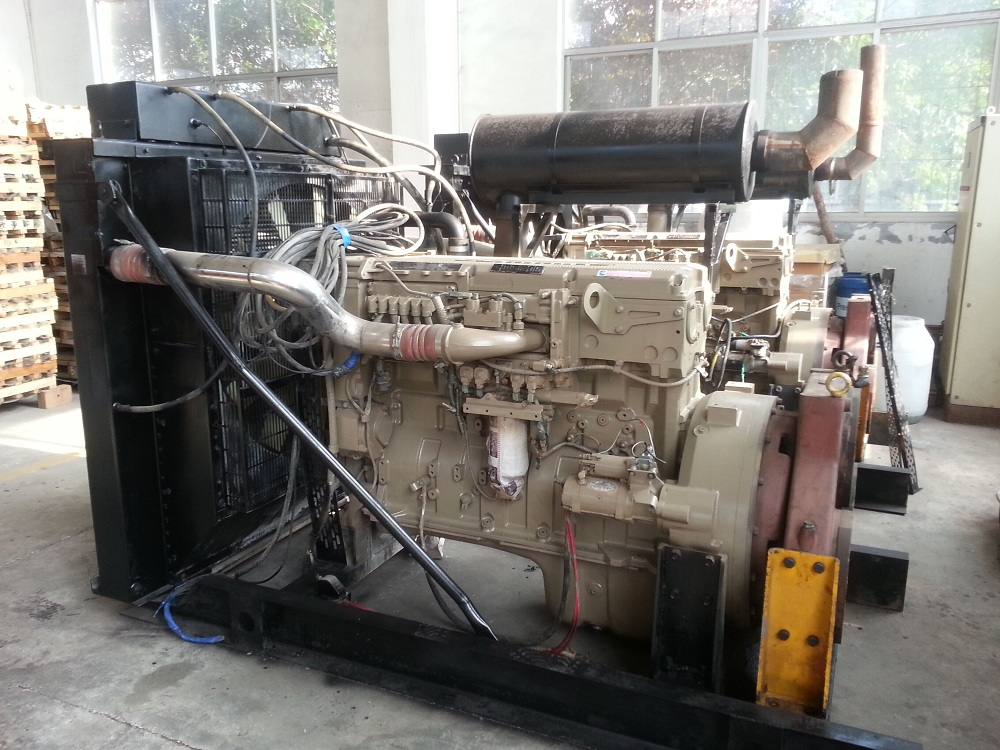# Reactive power limit of variable speed constant frequency wind turbine

In recent years, wind power generation in the world has developed rapidly, and wind power installed capacity is growing at an annual rate of more than 20%. By the end of 2002, the installed capacity of wind power generation in the world was about 31,128 ä¸½, of which China's wind power installed capacity reached 468.42 ä¸½. At present, the megawatt wind turbine has gradually replaced the 600kW class, becoming the main model of the international wind turbine market. The wind turbine is developing and improving in the direction of large-scale, variable pitch and variable-speed constant frequency.

Although the variable speed constant frequency wind turbine has a significant improvement in performance compared with the fixed speed wind turbine, the fund project due to wind speed change: National High Technology Research and Development Program (863 Program) (2001AA512021-5).

Randomness, the grid-connected operation of the variable-speed constant-frequency wind turbine is still a fluctuating impact power for the power system, so it is necessary to study the grid-connected operation characteristics of the wind turbine. The generator of the variable-speed constant-frequency wind turbine adopts the doubly-fed induction motor, and its steady-state model is studied. The mathematical model of the dq coordinate system based on the synchronous rotation with the stator magnetic field is established. Because the rotational speed of the doubly-fed generator and the reactive power on the stator side can be adjusted, the rotational speed control law and the reactive power control law also have a great influence on the steady-state characteristics of the variable-speed constant-frequency wind turbine. The basic idea of â€‹â€‹speed control and reactive power control is introduced. The goal of speed control is to optimize the power coefficient of the wind turbine, while the reactive power control can be set according to the actual operation mode of the power system to which it is connected. Two control modes: constant power factor or constant terminal voltage.

The active power generated by the wind turbine depends mainly on the wind speed, while the reactive power depends on the reactive power control scheme of the wind turbine. Generally, the wind farm is located in a remote area, and the power grid structure is weak. When the set value of the reactive power control reaches the reactive power limit of the wind turbine, on the one hand, the rotor winding heat will cause the wind turbine to stop, and on the other hand, it cannot be provided to the system. Or absorbing enough reactive power will cause the terminal voltage to decrease or rise, and in severe cases will cause the system voltage to be unstable. Therefore, it is necessary to study the reactive power limit of the variable speed constant frequency wind turbine. Some research has been done on this problem, but it only discusses the steady-state operating domain problem of active power and reactive power in the generator stator winding, and does not solve the steady state of active power and reactive power of the entire wind turbine injection system. Running domain issues. In addition, this does not consider the influence of the speed control law.

In this paper, the steady-state mathematical model of the variable-speed constant-frequency wind turbine is established. Based on this model, a method for calculating the reactive power limit is proposed. The method solves the active power of the wind turbine injection system and the steady-state operating domain of the grid technology reactive power. The problem, and considered the impact of the speed control.

2 Mathematical model of variable speed constant frequency wind turbine 2.1 The mechanical power of the wind turbine The constant frequency wind turbine adopts the doubly-fed induction motor. Compared with the fixed speed wind turbine, the variable frequency device between the stator winding and the rotor winding is added. The basic structure As shown.

The mechanical power of the wind turbine can be expressed as the proportion of the mechanical energy converted in the air kinetic energy, which is a function of the pitch angle and the tip speed ratio (2=, the ruler is the blade radius, which is the wind turbine speed); A is the blade sweep. Wind area; p is the air density; v is the wind speed. The steady-state equivalent circuit of the generator model doubly-fed induction motor is shown.

According to the steady-state mathematical model of the doubly-fed induction motor, the stator side power is determined by the powerful machine parameters for the rotor side power; opt is the coefficient determined by the blade parameters of the wind turbine, which can keep the wind turbine power coefficient optimal; Me is the lower limit of the speed of the wind turbine; max is the upper limit of the speed of the wind turbine; åŸ¤ is the speed of the wind turbine corresponding to the synchronous speed of the generator.

3 reactive power range of variable speed constant frequency wind turbines notebook3 P=198kW; P2=576kW; P3=656kW. Table 1 Example Wind power characteristics of variable speed constant frequency wind turbines The active power of wind turbine injection system is the active power of stator windings And the sum of the active power of the rotor windings. In the subsynchronous speed mode, the rotor winding absorbs the active power; in the super synchronous speed mode, the rotor winding emits active power. The active power of the rotor winding can be found by equation (8).

The stator side active power and reactive power operating range are affected by the current limit of the stator winding, the rotor winding and the rotor side of the converter, but the main function of the stator is the current limitation of the rotor side of the converter, which can be expressed as the wind turbine injection system. When the active power changes, its reactive power limit curve is as shown. It can be seen that the curve is not a regular curve. This is because when the active power Pe of the wind turbine injection system changes, the slip s will change according to the control law shown, then the parameters of the first equation in equation (10) will be As a result of the change, the curve changes irregularly. In the figure, the curve in the upper half plane represents the limit of the reactive power generated by the wind turbine, and the curve in the lower half plane represents the limit of the reactive power absorbed.

When the voltage at the end of the wind turbine changes, its reactive power limit also changes. The higher the terminal voltage, the lower the reactive power limit it emits and the greater the reactive power limit that can be absorbed.

The rated current is 150Â°%. Substituting equation (6) into equations (8) and (9) respectively, equation (10) is a space surface equation, and its projection in Pe-Qe plane is variable speed constant frequency wind turbine. The range of reactive power limits. When the active power Pe of the wind turbine is zero, the corresponding reactive power limit can be obtained from equation (10).

Select the variable speed constant frequency wind turbine with rated power of 800kW, the pressure is 690V; the rated power factor is -0.98. Its wind power characteristics are shown in Table 1. The generator speed range is -30% to +22%. The law is as shown, where =0.7 is called; 5 Conclusion According to the structure and working principle of the variable-speed constant-frequency wind turbine, the steady-state mathematical model of the variable-speed constant-frequency wind turbine is established, and a calculation of the variable-speed constant-frequency wind turbine is proposed. The method of reactive power limit, which can calculate the active power and reactive power operating range of the wind turbine injection system. The calculation and analysis of a 800kW variable-speed constant-frequency wind turbine are carried out by the method proposed in this paper. The calculation results show that the stator-side reactive power of the variable-speed constant-frequency wind turbine can be adjusted within a certain range, and the adjustment range is dependent on the stator-side active power. The change is affected by the rotor speed of the wind turbine and the power of the rotor side. After considering the speed control of the wind turbine, the boundary of the steady-state power operating range is an irregular curve of a random voltage change.

The method can be used to determine the reactive power control setting value of the variable speed constant frequency wind turbine. In addition, in the power system power flow calculation, the variable speed constant frequency wind turbine can be processed as a PV node, and the method can determine the reactive power limit of the variable speed constant frequency wind turbine. When the reactive power generated by the wind turbine exceeds the reactive power limit, its node properties will be converted from PV nodes to PQ nodes.

Diesel Engines

1. Diesel engines is A Machine which can turned other form energy into mechanical engergy . In base of diesel engine fuel matrerial , we can classfied diesel motor and gasoline engines. Diesel engine base techinal prometers is Engine displacement and Number of  cylinders. Engine cylinders has single cylinder and multi-cylinder diesel engines, Accoding cylinder arragament , we have Line engine and L type motors.NTA855 6 Cylinders Diesel Engines for Shantui SD22 bulldozer

2. Diesel engine assemly brand :

Engine Abroad : Cummins, Volvo, Caterpillar, Perkins, Deutz, Mitsubishi, Kubota, Yanmar, Isuzu Diesel Engines

Chinese engine : Weichai , Shangchai, Yuchai, Caochai diesel enginesIsuzu 6G1-TRP Diesel engines for 20T Exvavators

3. Diesel engine applications

Engine Motor has wide range useful ,  It is main use for :

-Generator/Genset - Silencer and Removable Generators

-Marine Equipment - Ship Machine and Ship Machine Auxiliary

-Mine Machinery Equipment - Heavy Trucks , Tipper, Dumper

-Electric Power Equipment - Power GenerationQSX15 Diesele engines for Power Generators

4. FAQ :

1. ) How to find Engine Nameplate ?

The Nameplate of Diesel engine is on the Engine gear housing or side of

Gear housing ,you can find and send ,we have professional engineer service

To you .

2. ) If we did not have parts nuber of diesel engine , how to solve ?

We have engine systerm to looking for your parts number and find the price

Of  Diesel engines and Engine Parts .

3. ) Which package for the diesel engines ?

Woodcase with Standard imported comfort long time  shipment .

4. ) How many days of your delivery time ?

7-10 working days from Qingdao or Tingjin port .

5. )Which Payment method you will accepted ?

T/T, Westerunion, Paypal,Bank account .

Diesel Engine Brand :

Diesel Engines: Cummins Diesel Engine, Weichai Diesel Engine, Shangchai Diesel Engine, Komatsu Diesel Engine, Yanmar Diesel Engine .

Diesel Engines

Cummins Diesel Engine,Marine Diesel Engine,Diesel Engines,Cummins Engines For Generator

Jining Kunpeng Construction Machinery Equipment Co., Ltd , https://www.excavatorenginepart.com

Posted on